## General Chemistry

Learn the toughest concepts covered in Chemistry with step-by-step video tutorials and practice problems by world-class tutors

17. Acid and Base Equilibrium

# pH and pOH

pH vs. pOH

The pH and pOH of a compound helps to determine its acidity and basicity.

The pH Scale
1
concept

## Understanding pH vs1m
Play a video:
Hey, guys, In this new video, we're gonna take a close look at P H and P O H. So we're gonna say that when it comes to the concentrations of H positive and O H minus, they usually come as very small numbers. And what we need to realize here is that the pH scale was invented to basically turn these varying small numbers into more manageable ones. So we're gonna stay under normal conditions. The pH scale operates within the range of zero tau 14. But what we need to realize here is that the PH scale could be less than zero, or it could be greater than 14. All we would need to do is increase the concentration of our acid or base. Remember, 0. 14 are not the only numbers that your pH your pH could be that could be greater or less than that. It all again depends on upping the concentration of your compound. If you make your concentration very high, like over one Moeller, then I'll give you a very high or very low pH. Now we're gonna say, by taking the negative log of H positive and O H minus, we confined Ph and P O h. So ph just means negative log of H plus concentration or negative log of h +30 plus. Because remember, we said this before H positive H 30 plus Same exact thing. P O. H is the negative log of O H minus. So that means that p represents negative log.

pH stands for the negative logarithmic function of your hydronium concentration, whereas pOH stands for the negative logarithmic function of hydroxide concentration.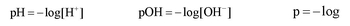2
concept

## The connection to Hydronium ion and Hydroxide ion concentrations1m
Play a video:
And what we should realize here is that by recognizing the relationship between h positive in O. H minus, we can find a new way to express them in terms of P H and P O H. So we come back here, we say that PH equals negative log of h positive. We could divide both sides by negative one to get rid of this negative. So negative pH equals log off h positive. Now we wanna just isolate th positive, so we're gonna divide out the log. When you divide anything by log, it becomes tend to that whatever the variable is. So we just find a new relationship. We can say that h positive. The concentration of H plus ions is equal to 10 to the negative pH. We could do the same thing with p. O. H. Divide both sides by negative one where negative p o. H equals log off O H minus divide both sides by log. So which minus equals 10 to the negative P o. H. So just remember the relationship we have here with these first two equations and how we have the relationship with their concentrations. Because sometimes you'll be asked to find HPE plus concentration. If they give you pH. Sometimes you may be asked to find O H minus concentration. If they give you p O H. You have to know the relationship both front words and backwards.

If we know the pH or pOH concentration then we can determine the concentration of hydronium ions or hydroxide ions.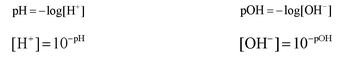3
concept

## The pH Scale2m
Play a video:
Now we're gonna say a species with a pH greater than seven greater than seven is classified as basic. If your basic you're gonna say that your H plus concentration is less than you're O h minus concentration. And we can also say that the stronger the base than the higher the pH and the higher the pH, the greater you're hydroxide concentration. Okay, so we can understand this relationship as strongest base would have the lowest pH I mean highest pH and therefore have the highest, all H minus concentration. Remember, we already went over this several videos ago. How do we determine if something is weak or strong? You still need to know those in order to do the following questions, because again, I've said it so many times before. Why do we need to know the strength of things? Because eventually we're gonna have to use ice charts to find pH if we're given a weak acid or a week base. That's why it's important to know what our species is is a week or is it strong? Now we're gonna say a species with a pH less than seven is termed acidic. And if you're acidic, your H plus concentration is greater than Euro H minus concentration. Here we can say the stronger the acid than the lower the pH. So strongest acid has the lowest pH, which means you have the highest h plus. And then finally, we're gonna say species with a pH equal to seven is neutral. Remember, this is Onley true at 25 degrees Celsius. Because, remember, at 25 degrees Celsius, K w equals 1.0 times 10 to the negative 14. If they were to change the temperature, that would change our K W. And then neutral Ph would become a new number. It doesn't always equal seven. And we're gonna stay here if you're neutral and your age plus concentration is equal to your O H minus concentration.

If the pH is less than 7 then the solution is acidic, if the pH is equal to 7 then the solution is neutral and if the pH is greater than 7 then the solution is basic.

4
concept

## The pH and pOH connection1m
Play a video:
and we're going to stay here. We talked about P H and P O. H and just realized their relationship is connected together by this equation, we're gonna say ph plus p o h equals 14. This arises by the fact that we have k w equals h positive times O H minus. And if you take the negative log off H plus, it gives you pH if you take the negative log off. O H minus. It gives you P O H and K W was 1.0 times 10 to the negative 14. And if you took the negative log of K W, it would give you 14. So that's where this equation comes from. It comes from taking the negative log of all of this. And remember, if you have two things multiplying like H positive and O. H minus are multiplying and you take the negative log of that whole thing, then it becomes the addition between them. Okay, so these guys are related. These two equations that I circled are related because they're connected together by negative long. So just remember that relationship and you'll be able to remember this formula. Remember? Ph scale 0 14 you could be below zero or higher than depending on the concentration of the acid or base you're using.

Under normal conditions when the concentration is less than 1.0 M the pH scale is between 0 to 14. pH and pOH are connected by the following equation: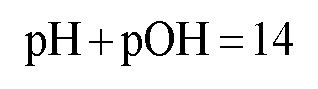5
example

## What is the hydroxide ion and hydronium ion concentration of an aqueous solution that has a pH equal to 6.123m
Play a video:
Hey, guys, In this new video, we're gonna put to practice some of the concepts we learned about P. H and P O H. So let's take a look at the first example here it says, What is the hydroxide ion? So what's the O. H minus and the hydrogen ion concentration or hide rhodium ion concentration. Actually, that's H plus often acquis solution that has a pH equal to 6.12. So we have pH we need to find O h minus and h plus. Remember, we're able to derive a formula that connects us between H plus and pH. Remember that H plus equals 10 to the negative pH. So I would have to do now is just take that number and plug it in. When we do that, we get 7.59 times 10 to the negative seven. Now, we could find ohh now, and we confined Ohh in two different ways. Now, the first way we could find o. H minus is by simply using this equation. Kw equals H plus times. Ohh! Minus we know what K W is. We assume the temperature is 25 degrees Celsius. Since they don't tell us what it is. So this will be 1.0 times 10 to the negative 14. We just found out. What h plus is it? 7.59 times 10 to the negative seven. Now we have to find O H minus. Ohh! Mind this is our only missing variable. So just isolated. So here, o H minus would equal 1.32 times 10 to the negative eight. So that would be one way we confined O h minus. Now, the second way we could find it is if we know p o. H. We could make a connection between p o H and O H minus. So, first we'd say that pH plus p o H equals 14. We know Ph is 6.12. So let's just isolate r p o h subtract 6 12 from both sides. So p o. H equals 7.88 Remember, what's the connection between P. O. H and O H minus? You could just simply say that oh h minus equals 10 to the negative P o. H. And if you did 10 to the negative 7.88 you get the same exact answer for O. H minus. So two different methods to find the same exact missing variable. So it's basically your choice on deciding which way you want to go. Personally, I like to just find P o H and just do 10 to the negative p o. H. Because remember, if you don't put these two in brackets, your calculator may give you the incorrect answer. So it's probably always safer just to say oh h minus equals 10 to the negative P O. H.
6
Problem

Which of the following solutions will have the lowest concentration of hydronium ions?

7
Problem

Which of the following statements about aqueous solutions is/are true?

8
example

## The pH Scale Example 24m
Play a video:
9
Problem

What is the Kw of pure water at 20.0°C, if the pH is 7.083?

Auto – Ionization Product
10
concept

## Understanding the Auto-Ionization Reaction3m
Play a video:
Hey, guys, In this new video, we're gonna take a look at the auto ionization of water. So what we're gonna say here is in an acquis solution. So the solvent is water. Water molecules can react with each other on. We're gonna say this is called the process off auto ionization or self ionization. Now, in this process, we're going to create H 30 plus and O H minus. Now just realize, how does this happen for this to occur? One of the water molecules has to act as an acid, and the other one has to act as a base. So let's say that this one here acts as the acid, and this year is the base. Remember, Bronston Laurie definitions. The acid acts as an H plus donor. The base acts as an H plus except er, so this base right here is going to accept an H plus, and as a result, it's gonna become H 30 plus. Then we're gonna say that the other water molecule, the one that's acting as an acid because it's giving away an H plus it becomes O. H minus and just also realize here that water molecules exists in liquid form. But then once they become these ions the hydro Nia, my on and the hydroxide ion they're gonna become a quiz. So they're no longer liquid water. And because of that, they're no longer a liquid. There gonna be a quiz? Remember, A quiz just means that the parts of the water are attracted to these particular ions. We can simplify auto ionization or self organization by just saying that water separates into H plus plus O H minus. Remember, we've said this before that h plus is the same thing as H 30 plus. Okay, so both are hydro Nia, my on They both mean the same exact thing. Now we're gonna say here that the equilibrium equation for water is called the ion product and I on product, it's simply said to bkw So when we're talking about the k w for water, we're talking about the ion product of water and we're gonna say that K w equals your hydro knee my on multiply times, your hydroxide ion, and we're gonna say here that at 25 degrees Celsius, K W is 1.0 times 10 to the negative 14. But remember, Just like all case, it's going to be temperature dependent. If you change the temperature, you're gonna change the value of the K W. So we're gonna say increasing the temperature will increase our kw. Now, here I give us a bunch of different values for K W, found at various temperatures and notice as a temperature. Gradually increases are K W will get bigger and bigger now. Just realize on your exam when you need to use K W. If they don't give you a temperature, just assume that it's 25 degrees Celsius. So then K W would be 1.0 times 10 to the negative 14. Now, knowing this, let's take a look at this question here.

In a self-ionization reaction two water molecules react with one another, where one acts an acid and the other as a base.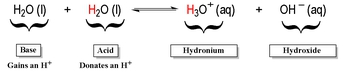The ionization product of Kw is used in this self-ionization equation and ignoring solids and liquids gives the  equilibrium equation as: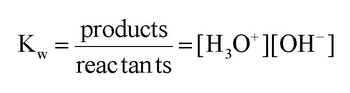11
example

## Determine the concentration of hydronium ions for a neutral solution at 25oC and at 50oC2m
Play a video:
So this question states determine the concentration of hydro Nia my on so basically find H plus or H +30 plus they both mean the same thing for a neutral solution at 25 degrees Celsius and 50 degrees Celsius. Okay, Now what we should realize here is what does neutral mean? Neutral means that your compound is not acidic or basic, and what it really means is that h 30 plus equals O H minus. They're both the same thing and because they're equal to each other, we're gonna set them both to an unknown variable. We're gonna set them two X and then what we're gonna do here is we know at 25 degrees Celsius, k w will be 1.0 times 10 to the negative 14. So a 25 degrees Celsius, That's what our K W. Is. And we just said that h 30 plus in which minus are equal when the solution is neutral and we're gonna set them both equal to X. So then you're gonna say x times X really means x squared and you only wanna find X, not X squared. So take the square root so in this case, X equals 1.0 times 10 to the negative seven. That number right there represents my concentration of H 30 plus. And technically, because it's neutral, it also represents the concentration of O H minus. Now, if you wanted to do it at 50 degrees Celsius would have to realize that since the temperature changes kw changes to this new number here. So we're gonna say 5.476 times 10 to the negative 14. Same exact process equals X squared. We just want X. So we're gonna take the square root of both sides. When we do that, it's going to give us a final answer of X equals 2. times 10 to the negative seven? No. Now So remember, this is important to know because K w equals H plus and times O H minus. This equation is important because of the temperature temperature is not given. Assume that K w equals 1.0 times 10 to the negative 14. If you know h plus concentration, then you know O h minus concentration. Because K w will be our constant and vice versa. If you know o h minus. You confined h Plus, this is the relationship we need to understand and these air the beginning steps for us to figure out the p H and p o h of different types of acquis solutions.
pH of Strong Acids and Bases

If we have a STRONG ACID or STRONG BASE then we DO NOT use an ICE chart to find the pH of solution because the yield of H+ and OH  ions are known.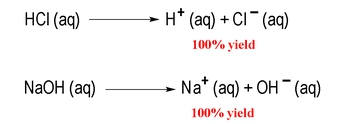12
concept

## pH of Strong Acids &amp1m
Play a video:
Hey, guys, In this new video, we're gonna take a look at the connection between Ph and P. O. H and how they relate to strong acids and strong bases. So remember, we've talked about this before. We're gonna say that strong acids and strong bases are considered to be strong electrolytes. Remember strong electrolytes, Completely ionized. That means they break up 100%. You may even hear that these strong acids and bases air called highly electrolytic. That also means they break up completely highly electro politic. So if we take a look here, we have HCL in N a o h. Remember, from the rules we've learned, we know HCL is a strong binary acid, and we know n a O. H is a strong base because we have a group one ai on and a positive connected to O. H minus. So what happens here? We have a single arrow going forward because they break up 100% to give us thes ions. We'd say that the product side is highly favored and just realize if we have a strong acid or a strong base, I've said it before. We don't have to use a nice chart in order to find pH or P O. H. So if you have a strong acid, take the negative log to find pH. If you have a strong base, take the negative log to find P O. H. So let's take a look. At example, one.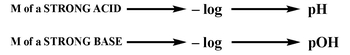13
example

## pH of Strong Acids &amp4m
Play a video:
so an example? One, it says calculate the pH off a 0.782 Moeller solution off. Calcium hydride. No, we know that calcium hydride is a strong base. Because remember, we have calcium, which is C A two plus connecting with h minus are hydride ions. So what happens here? The two from here came here. The one from here came here. That's how we got calcium hydride. And what we should realize here is that there are two calcium hydride in the formula. Now, this is what you need to remember. When it comes to strong basis for strong bases. You have to be very careful. We're going to say that this is not the real concentration of our strong base. You're gonna say when you have strong bases, you have to look at the number off O H minus H minus and H two minus 02 minus. So you have to look to see how many of each you have in order to determine the rial concentration off that strong base. In this particular question, we have to h minus is and because we have two of them, we have to multiply this by two that will give us the correct concentration off our strong base. Okay, so you have to remember that. So, for example, if I had C a o. H. Two and there was a 0.100 Moeller of it again because you have 20 H is you'd have to multiply that by two. If you have calcium of mind, you would have to an h two. So again, you have to multiply the concentration times to to get the correct concentration. Now, what we're gonna do is we're gonna multiply the initial concentration times to to get the rial concentration. Okay, When we do that, we're gonna get our correct concentration. That's the number that we're gonna plug in. So when we do that, and I'm gonna remove myself guys from the image so we have more room to work with When we do that, we're gonna get a new number a new value for r O. H. Minus concentration. So here, we're gonna get 0.1564 Mueller, that becomes our new concentration. So we're going to say p o. H equals negative. Log off O H minus. So it becomes negative. Log off 0.1564 Moeller. Now, some of you may be asking, but Jules, this is saying the concentration of O. H minus. We don't have O. H minus. We have h minus. How do they relate? Just realized that all four of these ions can be treated as being the same. They all feed into the concentration of O H minus. Okay, so h minus. Just understood as a general term for the strong basic ion off a strong base. So if I have to h 20 h minus is that's equivalent to two h minus is equivalent to two NH two minuses. Same thing. So when we take the negative log of this, this will give us our p o h. It's gonna give us 0.81 for our p o h. But remember, let's pay attention. I didn't ask for the pH I asked for. I didn't ask for the p o. H. I asked for the pH. So we need to use the next equation. PH plus p o h equals 14. Plug in what we know. For P o h. 0.81 we need ph. So subtract 0.81 from both sides. So ph here equals 13.19 So that will be our pH for this first question. Hopefully, guys didn't get lost. But what I was saying Just remember for strong bases you have to look to see how Maney o H minus is You have h minus is you have an H two minuses. You have an 02 businesses you have their number, determines the correct concentration of our strong base. And right now, what you need to realize is we only do this for strong base. At this point. If we had a strong acid such as H two s, 04 that strong acid has too acidic. H is. But we wouldn't need to multiply the concentration times too, because when it comes to acids, the first age coming off extremely strong. But the second one that comes off is extremely weak. And because the second H plus is extremely weak, we wouldn't need to multiply the concentration times too. So we only do this multiplication of concentrations when we have strong basis. Later on, when we deal with acid based titrate, shins and buffers, we'll talk about how assets play a role
14
example

## pH of Strong Acids &amp3m
Play a video:
15
Problem

Calculate the pH of 50.00 mL of 4.3 x 10-7 M H2SO4.

pH of Weak Acids and Bases

If we have a WEAK ACID or WEAK BASE then we DO use an ICE chart to find the pH of solution because the yield of H+ and OH  ions are unknown.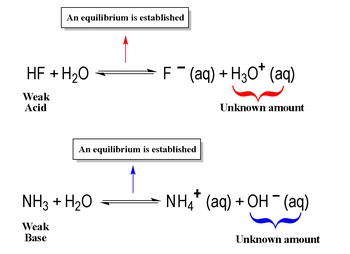16
concept

## Calculating the pH of a Weak Acid or Weak Base57s
Play a video:
Hey, guys, in this new video, we're gonna take a look at How exactly do we calculate the pH or P O. H of a weak species? So remember, we've talked about this before. We're gonna say that weak acids and bases are weak electrolytes, which means they don't completely break up when they dissolve in water. And what you should notice here is that we're gonna have double arrows because instead of going to completion, they're gonna establish in equilibrium and we're gonna say here for weak acids and weak bases, the react inside is highly favored, which means that our product not product but our compounds stay mostly as reactant. Very little of it breaks down to give us products. So we're gonna say the react inside is highly favored. So majority of our compound will stay as react. It's

To determine the pH of a weak acid or weak base we must use an ICE Chart.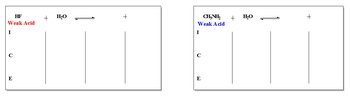17
example

## Pryridine, an organic molecule, is a very common weak base9m
Play a video:
18
example

## An unknown weak base has an initial concentration of 0.750 M with a pH of 8.03. Calculate its equilibrium base constant5m
Play a video: